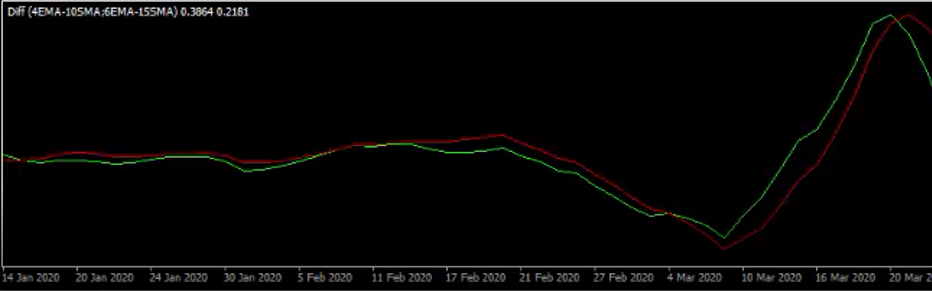# Plot differences between moving averages as lower indicator?

#### Hopperious

##### New member
1 line would be the difference of the 4EMA - 10SMA

the other line would be the difference of the 6EMA - 15SMA

used on any time frame --Try this very simple script...

Code:
``````#Plot lines with differences between two moving averages

declare lower;

input price = close;
input ema1 = 4;
input sma1 = 10;
input ema2 = 6;
input sma2 = 15;

plot line1 = ExpAverage(price, ema1) - Average(price, sma1);
plot line2 = ExpAverage(price, ema2) - Average(price, sma2);

line1.SetDefaultColor(Color.GREEN);
line2.SetDefaultColor(Color.RED);``````

•raymasa and ccurtin
tyvm - can we make this show an hour time frame but have it on lets say a 15min chart ?

tyvm - can we make this show an hour time frame but have it on lets say a 15min chart ?

This will only work on a time-based chart that is the same or smaller time-frame than the aggregation period but you can try this.

Code:
``````declare lower;

input Period = aggregationPeriod.HOUR;
input ema1 = 4;
input sma1 = 10;
input ema2 = 6;
input sma2 = 15;

plot line1 = ExpAverage(close(period = Period), ema1) - Average(close(period = Period), sma1);
plot line2 = ExpAverage(close(period = Period), ema2) - Average(close(period = Period), sma2);

line1.SetDefaultColor(Color.GREEN);
line2.SetDefaultColor(Color.RED);``````

•raymasa
Thanks for sharing, I realized this is actually close to something I want and that is way to tell how strong the difference is between a MA compared to recent history. This might help remove false-starts on MA (mostly MACD) indicators. I'm not quite sure what I'm looking for though. I can't just grab the median(x, length).

Code:
``````#Plot lines with differences between two moving averages

declare lower;

input price = close;
input sma1 = 9;
input sma2 = 26;

plot line1 = Average(price, sma1) - Average(price, sma2);
plot ZeroLine = 0;

plot Max = Highest(Average(price, sma1) - Average(price, sma2), sma2);

plot StrongUpSignal = if line1 equals Max
and line1 crosses Max
and price is greater than Zeroline
then ZeroLine else Double.NaN;

StrongUpSignal.SetDefaultColor(Color.UPTICK);
StrongUpSignal.SetPaintingStrategy(PaintingStrategy.ARROW_UP);

line1.SetDefaultColor(Color.GREEN);``````

Last edited:
•raymasa
Hello, just joined this forum, finding it very helpful. Thank you all. I tried the search but not able to find an answer to this question. In ToS, is there a way to scan for stocks where two moving averages are x% apart. For example, if EMA 8 is 10% above EMA 21? I am finding that EMA doesn't always cross to give a momentum indication. Sometimes, it comes close and bounced up (or down) again. Thanks

Thanks tradegeek and FoofandFun, I will try those and see how they perform.

### Not the exact question you're looking for?

87k+ Posts
169 Online## The Market Trading Game Changer

Join 2,500+ subscribers inside the useThinkScript VIP Membership Club
• Exclusive indicators
• Proven strategies & setups
• Private Discord community
• Exclusive members-only content
• 1 full year of unlimited support

What is useThinkScript?

useThinkScript is the #1 community of stock market investors using indicators and other tools to power their trading strategies. Traders of all skill levels use our forums to learn about scripting and indicators, help each other, and discover new ways to gain an edge in the markets.

How do I get started?

We get it. Our forum can be intimidating, if not overwhelming. With thousands of topics, tens of thousands of posts, our community has created an incredibly deep knowledge base for stock traders. No one can ever exhaust every resource provided on our site.

If you are new, or just looking for guidance, here are some helpful links to get you started.

What are the benefits of VIP Membership?